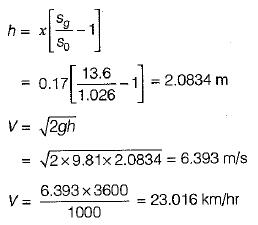Courses

# Test: Dynamic of Fluid Flow - 1

## 10 Questions MCQ Test Topicwise Question Bank for Mechanical Engineering | Test: Dynamic of Fluid Flow - 1

Description
This mock test of Test: Dynamic of Fluid Flow - 1 for Mechanical Engineering helps you for every Mechanical Engineering entrance exam. This contains 10 Multiple Choice Questions for Mechanical Engineering Test: Dynamic of Fluid Flow - 1 (mcq) to study with solutions a complete question bank. The solved questions answers in this Test: Dynamic of Fluid Flow - 1 quiz give you a good mix of easy questions and tough questions. Mechanical Engineering students definitely take this Test: Dynamic of Fluid Flow - 1 exercise for a better result in the exam. You can find other Test: Dynamic of Fluid Flow - 1 extra questions, long questions & short questions for Mechanical Engineering on EduRev as well by searching above.
QUESTION: 1

Solution:
QUESTION: 2

Solution:
QUESTION: 3

### Match List-I (Phenomenon) with List-ll (Equation/concept involved) and select the correct answer using the codes given below the lists: List-I A. Force developed in a bend B. Pitot-static tube C. Flow through smaller passage produces higher velocity D. Vortex flow List-ll 1. Continuity equation 2. Energy equation 3. Momentum equation 4. Moment of momentum equation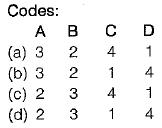Solution:
QUESTION: 4

The Bernoulli’s equation is written with usual  notation as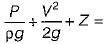constant . In this equation, each of the terms represents

Solution: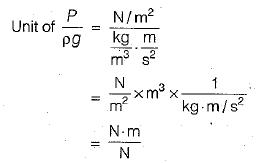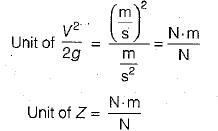QUESTION: 5

The difference between the total head line and the hydraulic grade line represents

Solution: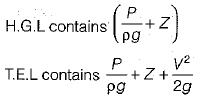QUESTION: 6

The dimension of kinetic energy correction factor α is

Solution:

Kinetic energy correction factor is basically defined as the ratio of kinetic energy of the flow per second based on actual velocity across a section to the kinetic energy of the flow per second based on average velocity across the same section.
Kinetic energy correction factor will be displayed by the symbol α and will be written mathematically as mentioned here.
Kinetic energy correction factor, α = (K.E. per second based on actual velocity) / (K.E. per second based on average velocity)

Hence, the dimensions are  M0L0 T0

QUESTION: 7

The kinetic energy correction factor a is defined as α =

where ,

V - average velocity in the cross-section

Solution:
QUESTION: 8

A 2-D conduit has the velocity distributions shown in figure below. What is kinetic energy correction factor? (Consider unit width of conduit)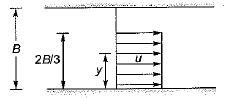Solution:

Discharge,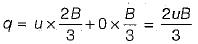Average velocity,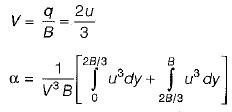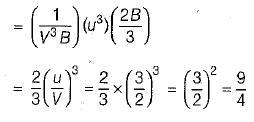QUESTION: 9

A pitot-static tube is used to measure the velocity of water in a pipe. The stagnation pressure head is 6 m and static pressure head is 5 m. The velocity of flow is [Take coefficient of velocity as 0.98]

Solution: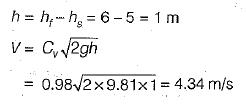QUESTION: 10

A sub-marine moves horizontally in sea and has its axis 15 m below the surface of water. A pitot- tube properly placed just in front of the sub-marine and along its axis is connected to the two limbs of a U-tube manometer containing mercury. The difference of mercury level is found to be 170 mm. What is the speed of the sub-marine knowing that the specific gravity of mercury is 13.6 and that of sea-water is 1.026 with respect to fresh water?

Solution: